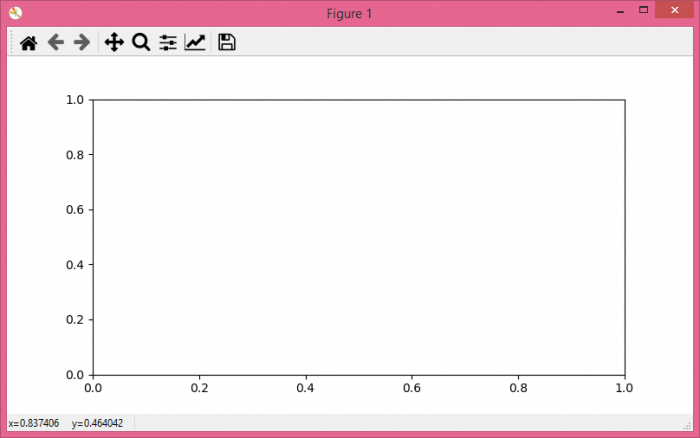# Interactive plotting with Python Matplotlib via command line

MatplotlibPythonData Visualization

#### Python Data Science basics with Numpy, Pandas and Matplotlib

Most Popular

63 Lectures 6 hours

#### Data Visualization using MatPlotLib & Seaborn

11 Lectures 4 hours

#### MatPlotLib with Python

9 Lectures 2.5 hours

To get interactive plots, we need to activate the figure. Using plt.ioff() and plt.ion(), we can perform interactive actions with a plot.

Open Ipython shell and enter the following commands on the shell.

## Example

In : %matplotlib auto
Using matplotlib backend: GTK3Agg

In : import matplotlib.pyplot as

In : fig, ax = plt.subplots() # Diagram will pop up. Let’s interact.

In : ln, = ax.plot(range(5))  # Drawing a line

In : ln.set_color("orange")   # Changing drawn line to orange

In : plt.ioff() # Stopped interaction

In : ln.set_color("red")
# Since we have stopped the interaction in the last step

In : plt.ion() # Started interaction

## Output